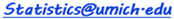Settings Controls
 ρ = μx= μy= σx= σy=
Use WebGL
 < X < < Y <
 Marginal of X Marginal of Y Conditional of X|Y = -∞ Conditional of X|Y = ∞ Conditional of Y|X = -∞ Conditional of Y|X = ∞
Probability Results
 Distribution graph Instructions Requirements - modern web-browser with HTML and JavaScript support. The 3D view of the bivariate distribution requires WebGL support. See the SOCR Bivariate Normal Distribution Activity. Use the Settings to initialize the web-app. To activate new input settings click outside the component. In the Control panel you can select the appropriate bivariate limits for the X and Y variables, choose desired Marginal or Conditional probability function, and view the 1D Normal Distribution graph. Use any non-numerical character to specify infinity (∞). You can rotate the bivariate normal distribution in 3D by clicking and dragging on the graph. Probability Results are reported in the bottom text area.
SOCR Resource Visitor number